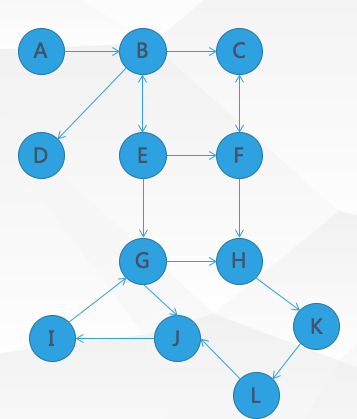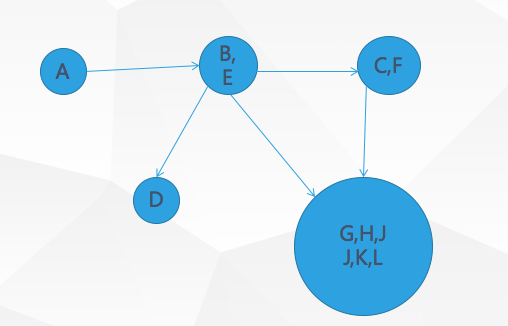<h1>概念</h1>

<h2>子图</h2>

<h2>强连通性</h2>

<h2>强连通分量（SCC）</h2>

<h1>Tarjan算法实现</h1>

Tarjan算法用于求有向图强连通分量，其过程主要如下：

DFS过程中，碰到哪个节点，就将哪个节点入栈。栈中节点只有在其所属的强连通分量已经全部求出时，才会出栈。

<h1>作用</h1><h1>例题</h1>

CodeVS 2822

<h2>题目描述</h2>

<h2>题解</h2>

<h2>代码</h2>

#include<cstdio>
#include<cstring>
#include<algorithm>
using namespace std;
const int N = 10003;
const int M = 50003;
void read(int &k) {
k = 0; int fh = 1; char c = getchar();
for(; c < '0' || c > '9'; c = getchar())
if (c == '-') fh = -1;
for(; c >= '0' && c <= '9'; c = getchar())
k = (k << 1) + (k << 3) + c - '0';
k = k * fh;
}

bool vis[N], inst[N];
struct node {int from, nxt, to;} E[M];
int DFN[N], low[N], point[N], cnt = 0, col[N], color = 0, st[N], top = 0, n, m, out[N], sum[N];
void tarjan(int x) {
DFN[x] = low[x] = ++cnt;
st[++top] = x; vis[x] = inst[x] = 1;
for(int tmp = point[x]; tmp; tmp = E[tmp].nxt)
if (!vis[E[tmp].to]) tarjan(E[tmp].to), low[x] = min(low[x], low[E[tmp].to]);
else if (inst[E[tmp].to]) low[x] = min(low[x], DFN[E[tmp].to]);
if (low[x] == DFN[x]) {
int u = 0; ++color;
while (u != x) {
u = st[top--];
col[u] = color;
inst[u] = 0;
++sum[color];
}
}
}
void ins(int x, int y) {E[++cnt] = (node) {x, point[x], y}; point[x] = cnt;}
int main() {
read(n); read(m);
int u, v;
for(int i = 1; i <= m; ++i) read(u), read(v), ins(u, v);
cnt = 0;
for(int i = 1; i <= n; ++i) if (!vis[i]) tarjan(i);
for(int i = 1; i <= m; ++i) {
u = col[E[i].from]; v = col[E[i].to];
if (u != v) ++out[u];
}
int ans = 0, tmp = 0;
for(int i = 1; i <= color; ++i) if (sum[i] > 1) ++tmp;
for(int i = 1; i <= color; ++i) {
if (out[i] == 0) {
if (ans != 0 || sum[i] == 1) {ans = 0; break;}
else ans = i;
}
}
printf("%dn", tmp);
if (ans == 0) puts("-1");
else {
for(int i = 1; i <= n; ++i)
if (col[i] == ans)
printf("%d ", i);
}
return 0;
}

Last modification：April 7th, 2020 at 10:14 pm